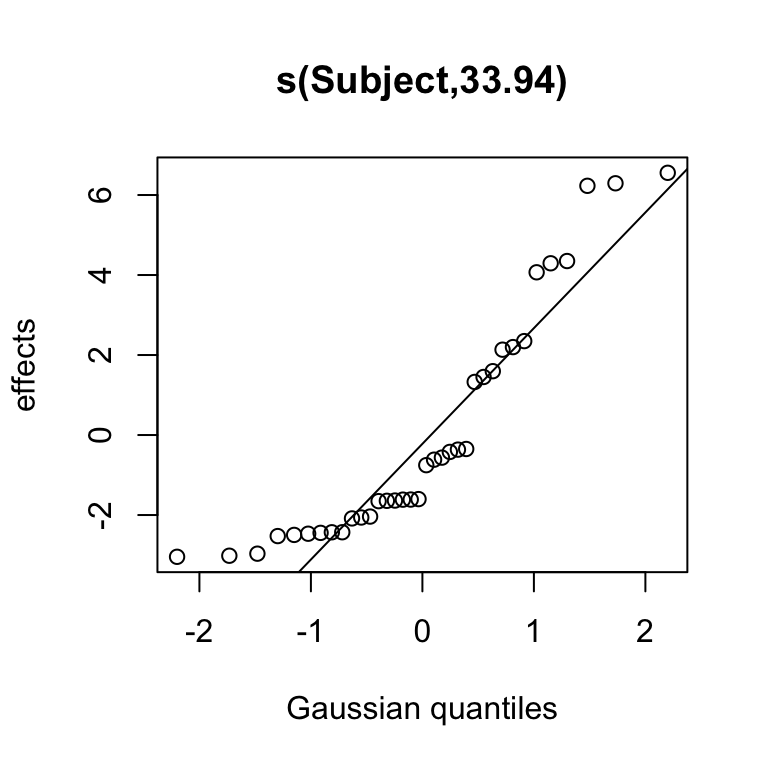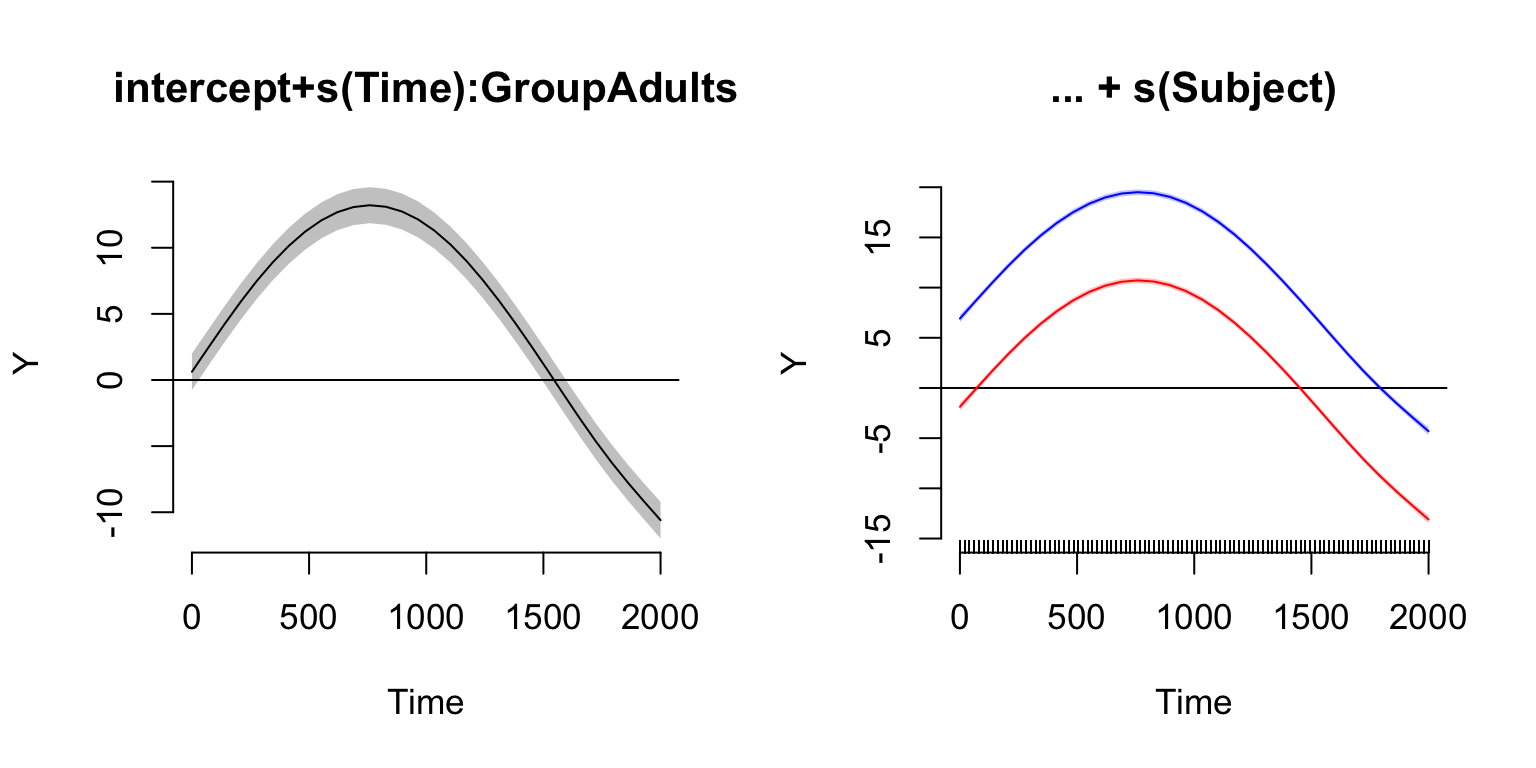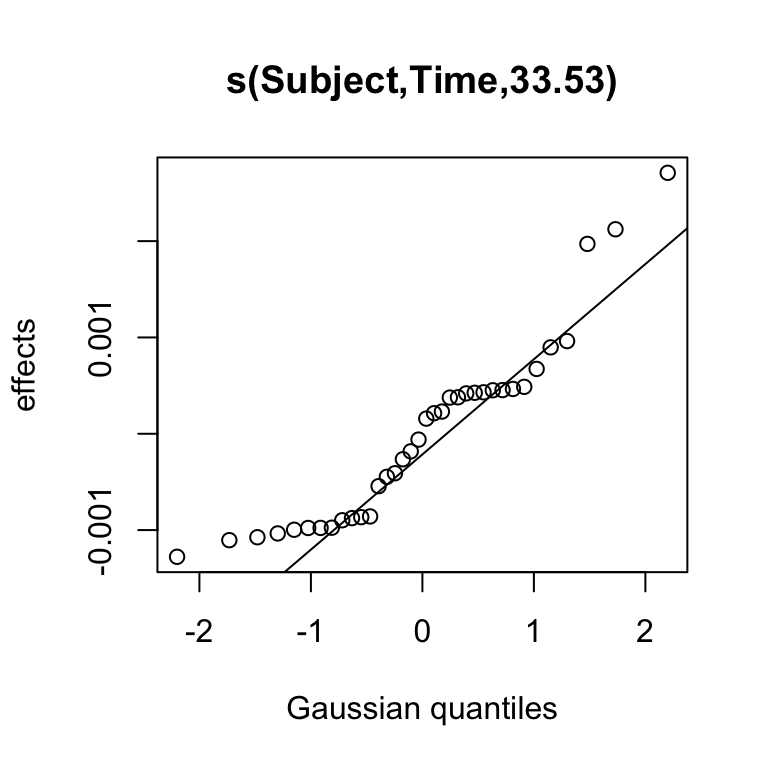Disclaimer: This short overview of a GAMM analysis is somewhat outdated, because some newer features of the packages mcgv and itsadug are not yet included. Note, however, that the basics (covered here) are still the same. I'm planning to update this summary, but in the meantime you may want to look at more recent analysis scripts here.

# Data and R packages

To give an overview of a GAMM analysis, we use the simulation data simdat of the package itsadug. First load the packages mgcv and itsadug.

## How to install the R packages

Both packages could be installed from CRAN using the commandline in R:

# installing packages from CRAN:
install.packages('mgcv')
install.packages('itsadug')

To install the newest version of itsadug directly from GitHub:

# installing the package devtools from CRAN:
install.packages('devtools')
library(devtools)
devtools::install_github("vr-vr/itsadug", build_vignettes=TRUE)

# itsadug automatically loads mgcv:
library(itsadug)
## Loading required package: mgcv
## This is mgcv 1.8-3. For overview type 'help("mgcv-package")'.
## Loaded package itsadug 0.92 (see 'help("itsadug")' ).

As the packages mgcv and itsadug are often updated, please check whether you have the latest version:

packageVersion('mgcv')
##  '1.8.3'
packageVersion('itsadug')
##  '0.92'

## Data

In this tutorial we use the simulated data simdat from itsadug:

data(simdat)
# inspect data:
str(simdat)
help(simdat)

However, you could also load your own data in R. If you have your data in Microsoft Excel, the easiest way is to export the data as .csv file:

File $$\rightarrow$$ Save As, then choose ‘Comma Separated Values (.csv)’.

dat <- read.table(file="mydata.csv", sep=",", header=TRUE)

If you did not use column names set the argument header to FALSE. The same function could be used to read a text file. The argument sep specifies the character being used to separate the columns. In text files this is often a tab, which could be specified with sep="\t".

# Analysis

For the current example, our question is whether adults and children reaction differently in their ‘Y’ response on the stimulus onset asynchrony, captured by the column Condition. Condition is manipulated between participants. The data set does not contain information about the different items, so for simplicity we assume that the experiment contained just one single item.

## gam() or bam()

There are two functions for implementing a GAMM model: gam() and bam(). There are largely similar. The most important difference is that bam() is optimized for big data sets (e.g., more than 10.000 data points)

nrow(simdat)
##  75600

## Smooths terms

To model a potentially nonlinear smooth or surface, three different smooth functions are available:
• s() :

• for modeling a 1-dimensional smooth, or
• for modeling isotropic interactions (variables are measured in same units and on same scale)
• te(): for modeling 2- or n-dimensional interaction surfaces of variables that are not isotropic (but see info about d parameter below). Includes ‘main’ effects.

• ti(): for modeling 2- or n-dimensional interaction surfaces that do not include the ‘main effects’.

Example models:

# 1-dimensional smooth of Time:
m1 <- bam(Y ~ s(Time), data=simdat)
# interaction with non-isotropicterms:
m2 <- bam(Y ~ te(Time, Trial), data=simdat)
# decompose the same interaction in main effects + interaction:
# (note: include main effects)
m3 <- bam(Y ~ s(Time) + s(Trial) + ti(Time, Trial), data=simdat)

## Parameters of smooth functions

The smooth functions have several parameters that could be set to change their behavior. The most often-used parameters are listed here:

• k : number of ‘knots’. This parameter determines the upper bound of the number of underlying base functions being used to build up the curve. Thus, this parameter constraints the wigglyness of a smooth, or - as a metaphor - the number of bowpoints of a curve.
• Note that the model will base the number of base functions (reflected in the edf of the summary) on the data with the setting for k as upper bound. By default, the value of k for s() is around 9, and for te() and ti() 5 per dimension. Importantly, the value of k should be at most one less than the number of unique data points, otherwise it will fit the density of that predictor.
• d : for specifiying that predictors in the interaction are on the same scale or dimension (only used in te() and ti()). For example, in te(Time, width, height, d=c(1,2)), with width and height reflecting the picture size measured in pixels, we specify that Time is on a different dimension than the next two variables. By default, the value would be d=c(1,1,1) in this case.

• bs: specifies the type of underlying base functios. For s() this defaults to "tp" (thin plate regression spline) and for te() and ti() this defaults to "cr" (cubic regression spline). For random intercepts and linear random slopes use bs="re", but for random smooths use bs="fs" (see below).

## Different types of interactions

For modeling potentially different trends over time for different age groups (CHildren, Adults), the by argument in the smooth functions could be used.

How to set up the interaction depends on the type of grouping predictor:

• with factor include intercept difference: Group + s(Time, by=Group).

• with ordered factor include intercept difference and reference smooth: Group + s(Time) + s(Time, by=Group).

• with binary predictor include reference smooth: s(Time) + s(Time, by=IsGroupChildren).

An intercept term needs to be included when using factors and ordered factors, because smooths with factors are centered. Including the intercept terms provides more flexibility for the model to fit overall intercept differences between factor levels, and avoids artifacts due to the centering constraints. Binary predictors are modeled as separate smooths, and hence are not centered around zero. As a result, binary predictors may yield different estimates that factors and ordered factors.

### Example factor:

# make predictor as factor:
simdat$Group <- as.factor(simdat$Group)
# inspect contrasts:
contrasts(simdat$Group) ## Adults ## Children 0 ## Adults 1 # change reference level: Adults is reference simdat$Group <- relevel(simdat$Group, ref="Adults") # inspect contrasts: contrasts(simdat$Group)
##          Children
## Children        1
# run model:
m.factor <- bam(Y ~ Group + s(Time, by=Group), data=simdat)
# inspect summary:
summary(m.factor)
##
## Family: gaussian
##
## Formula:
## Y ~ Group + s(Time, by = Group)
##
## Parametric coefficients:
##               Estimate Std. Error t value Pr(>|t|)
## (Intercept)    5.26277    0.03241  162.39   <2e-16 ***
## GroupChildren -3.18626    0.04583  -69.52   <2e-16 ***
## ---
## Signif. codes:  0 '***' 0.001 '**' 0.01 '*' 0.05 '.' 0.1 ' ' 1
##
## Approximate significance of smooth terms:
##                         edf Ref.df    F p-value
## s(Time):GroupAdults   8.586  8.951 5367  <2e-16 ***
## s(Time):GroupChildren 8.124  8.793 2924  <2e-16 ***
## ---
## Signif. codes:  0 '***' 0.001 '**' 0.01 '*' 0.05 '.' 0.1 ' ' 1
##
## R-sq.(adj) =  0.509   Deviance explained =   51%
## fREML = 2.4646e+05  Scale est. = 39.69     n = 75600

The summary of m.factor shows:

• Intercept adjustment for Children in comparison with Adults in parametric summary.

• The smooth terms summary shows two smooths that are significantly different from 0. We cannot conclude from the summary that the two curves are different from each other.

### Example ordered factor:

simdat$OFGroup <- as.factor(simdat$Group)
# change factor to ordered factor:
simdat$OFGroup <- as.ordered(simdat$OFGroup)
# change contrast to treatment coding (difference curves)
contrasts(simdat$OFGroup) <- 'contr.treatment' # Inspect contrasts: contrasts(simdat$OFGroup)
##          Children
## Children        1
# run model:
m.orderedfactor <- bam(Y ~ OFGroup + s(Time) + s(Time, by=OFGroup), data=simdat)
# inspect summary:
summary(m.orderedfactor)
##
## Family: gaussian
##
## Formula:
## Y ~ OFGroup + s(Time) + s(Time, by = OFGroup)
##
## Parametric coefficients:
##                 Estimate Std. Error t value Pr(>|t|)
## (Intercept)      5.26276    0.03241  162.39   <2e-16 ***
## OFGroupChildren -3.18621    0.04583  -69.52   <2e-16 ***
## ---
## Signif. codes:  0 '***' 0.001 '**' 0.01 '*' 0.05 '.' 0.1 ' ' 1
##
## Approximate significance of smooth terms:
##                           edf Ref.df    F p-value
## s(Time)                 8.662  8.926 5382  <2e-16 ***
## s(Time):OFGroupChildren 7.408  8.347  595  <2e-16 ***
## ---
## Signif. codes:  0 '***' 0.001 '**' 0.01 '*' 0.05 '.' 0.1 ' ' 1
##
## R-sq.(adj) =  0.509   Deviance explained =   51%
## fREML = 2.4646e+05  Scale est. = 39.689    n = 75600

The summary of m.orderedfactor shows:

• Intercept adjustment for Children in comparison with Adults in parametric summary.

• The smooth terms summary shows two smooths that are significantly different from 0: the reference smooth (for the Adults) and the difference smooth (Children - Adults). When the difference smooth is significantly different from 0, we can conclude that the two groups follow a different trend over Time.

### Example binary:

# create binary predictor:
simdat$IsChildren <- with(simdat, ifelse(Group=="Children", 1, 0)) # run model: m.binary <- bam(Y ~ s(Time) + s(Time, by=IsChildren), data=simdat) # inspect summary: summary(m.binary) ## ## Family: gaussian ## Link function: identity ## ## Formula: ## Y ~ s(Time) + s(Time, by = IsChildren) ## ## Parametric coefficients: ## Estimate Std. Error t value Pr(>|t|) ## (Intercept) 5.26264 0.03241 162.4 <2e-16 *** ## --- ## Signif. codes: 0 '***' 0.001 '**' 0.01 '*' 0.05 '.' 0.1 ' ' 1 ## ## Approximate significance of smooth terms: ## edf Ref.df F p-value ## s(Time) 8.663 8.926 5382 <2e-16 *** ## s(Time):IsChildren 8.408 9.347 1040 <2e-16 *** ## --- ## Signif. codes: 0 '***' 0.001 '**' 0.01 '*' 0.05 '.' 0.1 ' ' 1 ## ## R-sq.(adj) = 0.509 Deviance explained = 51% ## fREML = 2.4646e+05 Scale est. = 39.689 n = 75600 The summary of m.binary shows: • The smooth terms summary shows two smooths that are significantly different from 0: the reference smooth (for the Adults) and the difference smooth (Children - Adults). When the difference smooth is significantly different from 0, we can conclude that the two groups follow a different trend over Time. However, we do not know whether the trend over time is different, or whether the groups are overall showing a difference (intercept difference). ## Random effects Three different types of random effects are distinghuished when using GAMMs: • random intercepts adjust the height of other modelterms with a constant value: s(Subject, bs="re"). • random slopes adjust the slope ofthe trend of a numeric predictor: s(Subject, Time, bs="re"). • random smooths adjust the trend of a numeric predictor in a nonlinear way: s(Time, Subject, bs="fs", m=1). Notes: • Random intercepts and random slopes could be combined, but the random smooths already include random intercepts and random slope effects. • The argument m=1 sets a heavier penalty for the smooth moving away from 0, causing shrinkage to the mean. ### Example of random intercept: # first make sure Subject is a factor: simdat$Subject <- as.factor(simdat$Subject) # run model with random intercept: m1 <- bam(Y ~ Group + s(Time, by=Group) + s(Subject, bs="re"), data=simdat) summary(m1) ## ## Family: gaussian ## Link function: identity ## ## Formula: ## Y ~ Group + s(Time, by = Group) + s(Subject, bs = "re") ## ## Parametric coefficients: ## Estimate Std. Error t value Pr(>|t|) ## (Intercept) 5.2614 0.6903 7.621 2.54e-14 *** ## GroupChildren -3.1895 0.9763 -3.267 0.00109 ** ## --- ## Signif. codes: 0 '***' 0.001 '**' 0.01 '*' 0.05 '.' 0.1 ' ' 1 ## ## Approximate significance of smooth terms: ## edf Ref.df F p-value ## s(Time):GroupAdults 8.66 8.966 6730 <2e-16 *** ## s(Time):GroupChildren 8.26 8.850 3649 <2e-16 *** ## s(Subject) 33.94 34.000 569 <2e-16 *** ## --- ## Signif. codes: 0 '***' 0.001 '**' 0.01 '*' 0.05 '.' 0.1 ' ' 1 ## ## R-sq.(adj) = 0.609 Deviance explained = 61% ## fREML = 2.3795e+05 Scale est. = 31.601 n = 75600 Plot of distribution of random intercepts: plot(m1, select=3)Plot the summed effects for the adults without random effects (Left), and for two specific subjects (Right): # specify two plot panels: par(mfrow=c(1,2), cex=1.1) # summed effects without random effects: # This is actually: intercept + s(Time):GroupAdults plot_smooth(m1, view="Time", cond=list(Group="Adults"), rm.ranef=TRUE, main="intercept+s(Time):GroupAdults", rug=FALSE) # Plot summed effects for Subject "a01": plot_smooth(m1, view="Time", cond=list(Group="Adults", Subject="a01"), main="... + s(Subject)", col='red', ylim=c(-15,20), rug=FALSE) # Add Subject "a18": plot_smooth(m1, view="Time", cond=list(Group="Adults", Subject="a18"), add=TRUE, col='blue')### Example of random slope: # first make sure Subject is a factor: simdat$Subject <- as.factor(simdat\$Subject)
# run model with random intercept:
m2 <- bam(Y ~ Group + s(Time, by=Group)
+ s(Subject, Time, bs="re"), data=simdat)
summary(m2)
##
## Family: gaussian
##
## Formula:
## Y ~ Group + s(Time, by = Group) + s(Subject, Time, bs = "re")
##
## Parametric coefficients:
##               Estimate Std. Error t value Pr(>|t|)
## (Intercept)     5.2613     0.2345  22.436   <2e-16 ***
## GroupChildren  -3.1895     0.3316  -9.617   <2e-16 ***
## ---
## Signif. codes:  0 '***' 0.001 '**' 0.01 '*' 0.05 '.' 0.1 ' ' 1
##
## Approximate significance of smooth terms:
##                          edf Ref.df       F p-value
## s(Time):GroupAdults    8.597  8.953 3338.56  <2e-16 ***
## s(Time):GroupChildren  8.144  8.802  729.72  <2e-16 ***
## s(Subject,Time)       33.527 34.000   70.92  <2e-16 ***
## ---
## Signif. codes:  0 '***' 0.001 '**' 0.01 '*' 0.05 '.' 0.1 ' ' 1
##
## R-sq.(adj) =  0.525   Deviance explained = 52.5%
## fREML = 2.4534e+05  Scale est. = 38.463    n = 75600

Plot of distribution of random intercepts:

plot(m2, select=3)Plot the summed effects for the adults without random effects (Left), and for two specific subjects (Right):

# specify two plot panels:
par(mfrow=c(1,2), cex=1.1)
# summed effects without random effects:
# This is actually: intercept + s(Time):GroupAdults
add=TRUE, col='blue')